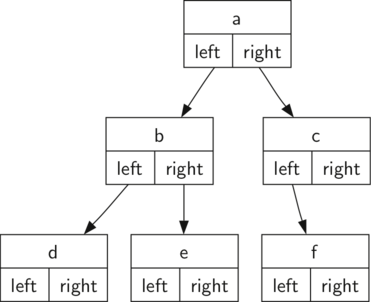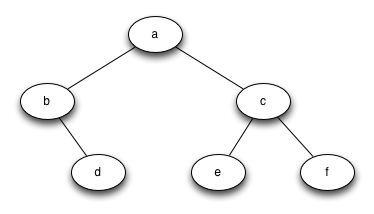# 7.5. Nodes and References¶

Our second method to represent a tree uses nodes and references. In this case we will define a class that has attributes for the root value, as well as the left and right subtrees. Since this representation more closely follows the object-oriented programming paradigm, we will continue to use this representation for the remainder of the chapter.

Using nodes and references, we might think of the tree as being structured like the one shown in Figure 2.Figure 2: A Simple Tree Using a Nodes and References Approach¶

We will start out with a simple class definition for the nodes and references approach as shown in Listing 4. The important thing to remember about this representation is that the attributes `left` and `right` will become references to other instances of the `BinaryTree` class. For example, when we insert a new left child into the tree we create another instance of `BinaryTree` and modify `self.leftChild` in the root to reference the new tree.

Listing 4

```class BinaryTree:
def __init__(self,rootObj):
self.key = rootObj
self.leftChild = None
self.rightChild = None
```

Notice that in Listing 4, the constructor function expects to get some kind of object to store in the root. Just like you can store any object you like in a list, the root object of a tree can be a reference to any object. For our early examples, we will store the name of the node as the root value. Using nodes and references to represent the tree in Figure 2, we would create six instances of the BinaryTree class.

Next let’s look at the functions we need to build the tree beyond the root node. To add a left child to the tree, we will create a new binary tree object and set the `left` attribute of the root to refer to this new object. The code for `insertLeft` is shown in Listing 5.

Listing 5

```1def insertLeft(self,newNode):
2    if self.leftChild == None:
3        self.leftChild = BinaryTree(newNode)
4    else:
5        t = BinaryTree(newNode)
6        t.leftChild = self.leftChild
7        self.leftChild = t
```

We must consider two cases for insertion. The first case is characterized by a node with no existing left child. When there is no left child, simply add a node to the tree. The second case is characterized by a node with an existing left child. In the second case, we insert a node and push the existing child down one level in the tree. The second case is handled by the `else` statement on line 4 of Listing 5.

The code for `insertRight` must consider a symmetric set of cases. There will either be no right child, or we must insert the node between the root and an existing right child. The insertion code is shown in Listing 6.

Listing 6

```def insertRight(self,newNode):
if self.rightChild == None:
self.rightChild = BinaryTree(newNode)
else:
t = BinaryTree(newNode)
t.rightChild = self.rightChild
self.rightChild = t
```

To round out the definition for a simple binary tree data structure, we will write accessor methods (see Listing 7) for the left and right children, as well as the root values.

Listing 7

```def getRightChild(self):
return self.rightChild

def getLeftChild(self):
return self.leftChild

def setRootVal(self,obj):
self.key = obj

def getRootVal(self):
return self.key
```

Now that we have all the pieces to create and manipulate a binary tree, let’s use them to check on the structure a bit more. Let’s make a simple tree with node a as the root, and add nodes b and c as children. ActiveCode 1 creates the tree and looks at the some of the values stored in `key`, `left`, and `right`. Notice that both the left and right children of the root are themselves distinct instances of the `BinaryTree` class. As we said in our original recursive definition for a tree, this allows us to treat any child of a binary tree as a binary tree itself.

Self Check

Write a function `buildTree` that returns a tree using the nodes and references implementation that looks like this: